# Light

### Reflection of Light

Some objects such as sun, stars, burning candles, lamp, etc. which emit light by their own are called luminous objects. Some other objects may bounce back a part of the light falling on them from any luminous object. This bouncing back of light after falling on any surface is called reflection of light.

When a beam of light comes in contact with an object, a part or all of it gets bounced back. This phenomenon is called reflection of light. Some objects having smooth and shiny surface reflect better than others. A smooth shining surface, which reflects most of the light incident on it, is called a mirror.The direction of propagation of light, a beam of light consists of number of rays. The incident ray is the ray of light falling on the reflecting surface. The normal is the line drawn at 90° to the surface at the point where the incident ray strikes the surface. The light coming back from the reflecting surface is called reflected ray. The angle of incidence is the angle between incident ray and normal and angle of reflection is the angle between reflected ray and normal.

### Laws of Reflection

The reflection of light from the surface takes place according to the following two laws:

1. Incident ray, reflected ray and the normal at the point of incidence, all lie in the same plane.
2. The angle of incidence is equal to the angle of reflection: ∠i = ∠r

During reflection, there is no change in speed, frequency and wavelength of light. Reflection of light may be classified as regular reflection and diffused reflection.

Regular Reflection

When reflecting surface is very smooth and the rays of light falling on it are reflected straight off it, then it is called regular reflection

Diffused Reflection

When the reflection of light takes place from rough surface the light is reflected off in all directions is called diffused reflection.

### Formation of Images

When an object is placed infront of a mirror its image is formed by reflection. Every point on the object acts like a point source, from which a number of rays originate. In order to locate the image of the point object, an arbitrarily large number of rays emanating from the point object can be considered. However, for the sake of simplicity, take any two rays of light (starting from the point object).

The paths on reflection from the mirror (reflected rays corresponding to the incident rays) are traced using laws of reflection. The point where these two rays actually meet is the real image of the point object.

If these rays appear to come from and not actually coming, the virtual image of the point is formed. Real images obtained by actual intersection of reflected rays, hence they can be projected on screen. Virtual images are obtained when the rays appear to meet each other but actually do not intersect each other, hence they cannot be cast on screen.

### Reflection at Spherical Mirrors

A spherical mirror is a section of a hollow sphere whose inner or outer surface is polished. There are mainly two types of spherical mirrors:

Convex Mirror

It is a mirror in which the reflection takes place from the bulging surface (inner side is painted and reflected surface is polished to make the surface smooth).

Concave Mirror

It is a mirror in which the reflection takes place from the cave side surface (outer side is painted and the inner or cave side surface is polished to make the reflected surface smooth).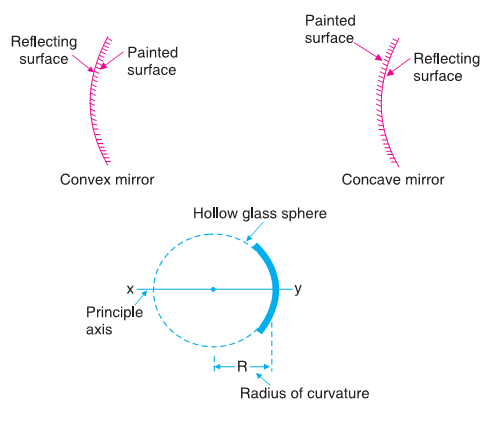Pole (P)

It is the mid point of the spherical mirror.

Centre of curvature (C)

It is the centre of a hollow sphere of which the spherical mirror is a part. It can be determined by finding the point of intersection of two normal drawn at the spherical surface of the mirror.

It is the distance between the pole and centre of curvature of the mirror.

Principal axis

It is an imaginary line joining the pole to the centre of curvature.

Principal focus (F)

The rays of light parallel and close to the principal axis of the mirror after reflection, either pass through a point (in concave mirror) or appear to be coming from a point (in convex mirror) on the principal axis; this point is called principal focus of the mirror.

Focal length (f)

It is the distance between the pole and the principal focus of the mirror.

### Relation Between f and R

Consider the reflection of light of ray IM at M at a concave mirror. CM is the normal drawn at the surface which passes through centre of curvature and MF is the reflected ray which passes through the focal point.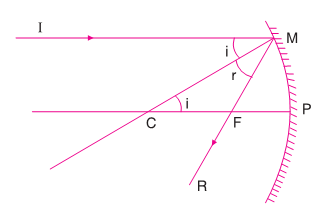Angle of incidence and reflection are equal.

∠i = ∠r

In ΔCMF, MF = CF

For small aperture of the mirror,

MF = PF

PC = PF + CF = PF + PF = 2PF

R = 2f

### Rules for Image Formation

The ray diagram for image formation by mirrors can be drawn by taking any two of the following rays. The point where these two rays meet or appear to be coming from the point will be the image point which determines the position of image.

1. Ray striking the pole: The ray of light striking the pole of the mirror at an angle is reflected back at the same angle on the other side of the principal axis.
2. Parallel ray: For concave mirror the ray parallel to the principal axis is reflected in such a way that after reflection it passes through the principal focus. But for a convex mirror the parallel ray is so reflected that it appears to come from principal focus.
3. Ray through centre of curvature: A ray passing through the centre of curvature hits the mirror along the direction of the normal to the mirror at that point and retraces its path after reflection.
4. Ray through focus: A ray of light heading lowards the focus or incident on the mirror after passing through the focus returns parallel to the principal axis.### Images by Concave Mirror### Image by Convex Mirror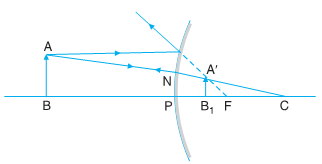The position, nature and size of the image formed in concave mirror and convex mirror can be summarized as:

 S.No. Object Position Image Position Nature Size Concave Mirror 1 Betwwen P & F Behind the mirror Virtual Larger 2 At F At infinity Real Enlarged 3 Between F & 2F Beyond 2F Real Larger 4 At 2F At 2F Real Same size 5 Beyond 2F Between F & 2F Real Smaller 6 At infinity At F Real Diminished Convex Mirror 7 Anywhere Between P & F Virtual Smaller

### Uses of Mirrors

Plane mirror

• in looking glasses
• in construction of kaleidoscope, telescope, sextant, and periscope
• for seeing round the corners
• as deflector of light

Concave minor

• as a reflector in searchlight, head light of motor cars and projectors
• for converging solar radiation in solar cookers
• in flood lights to obtain a divergent beam of light to illuminate buildings
• in reflecting telescopes

Convex mirror

• as a rear view mirror in motor cars, buses and scooters
• as safety viewers at dangerous corners and on upper deck of double decker buses

### Sign Convention

To measure distances with respect to a curved mirror, following convention is followed:

1. All distances are measured from the pole of the mirror.
2. The distances measured in the direction of incident light, are taken as positive.
3. The distances measured in opposite direction of incident light, are taken as negative.
4. The distances above the principal axis are taken positive, whereas those below it are taken as negative.

### Mirror Formula

In concave mirror, when an object is placed at 2f (centre of curvature) the image is formed at 2f. If f be the focal length of the concave mirror, u distance of object and v the distance of image, then

u = -2f

v = -2f

1/f = 1/-2f + 1/-2f

1/f = 1/v + 1/u

This is called mirror formula.

### Magnification in Spherical Mirrors

A spherical mirror can produce magnified image of an object. The ratio of the size of the image to the size of the object is called linear magnification (M).

M = size of image / size of object = I/O = v/u

### Refraction

When light passes from denser medium to rarer medium it bends away from the normal. When it passes from rarer medium to denser medium it bends towards the normal. This phenomenon of bending of light is called refraction of light.

Refractive Index of the Medium

When light travels from one medium to another its speed changes. A ray of light from a rarer medium to a denser medium slows down and bends towards the normal. On the other hand the ray of light going from a denser medium to a rarer medium is speeded up and bends away from the normal.

It shows that the speed of light in different medium varies. Different medium have different abilities to bend or refract light. This bending ability of a medium is known as the index of refraction or refractive index. It is defined as the ratio of the speed of light in vacuum to that in the material medium.

### Laws of Refraction

The extent, to which a ray bends, depends not only on the refractive index of medium, but also on the angle of incidence. The laws of refraction are:

First law of refraction: The incident ray, refracted ray and the normal at the point of incidence, all lie in the same plane.

Second law of refraction: How much ray of light refracted depends on that medium. The ratio of the sine of the angle of incidence to the sine of the angle of refraction is constant and equal to the refractive index of that medium. This law is also called Snell’s law.

Refractive index (n) = Sine of angle of incidence / Sine of angle of refraction

n =  sin i / sin r

### Refraction through Spherical Surface

A lens is a portion of a transparent refracting medium bounded by two surfaces. Depending upon the nature of surfaces lens may be of following types: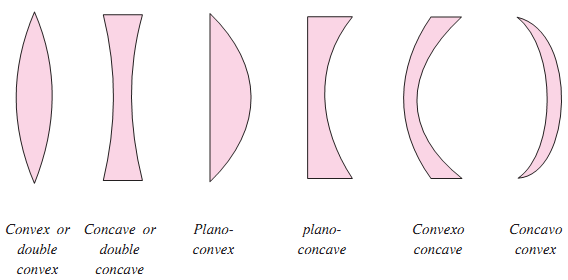Convex Lens

Convex lens has its two surfaces bulging outward. It makes the parallel rays of light to converge to a point. Hence, it is called converging lens. The point of convergence is called focus.Concave Lens

A concave lens has its two surfaces caving inward. It makes parallel rays of light to spread from a point. Hence, it called diverging lens. The point where from light rays appear to diverge is called focus.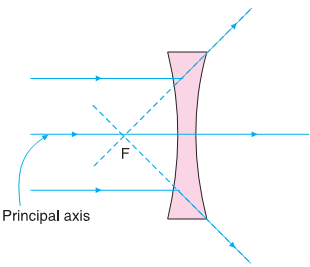### Image Formation in Lens

In order to draw the image formed by any lens, only two rays are required:

1. A ray parallel to the principal axis of the lens converges after refraction at the principal focus of convex lens. It appears to diverge off in the case of concave lens.
2. A ray towards the optical centre falls on the lens symmetrically and after refraction passes through it undeviated.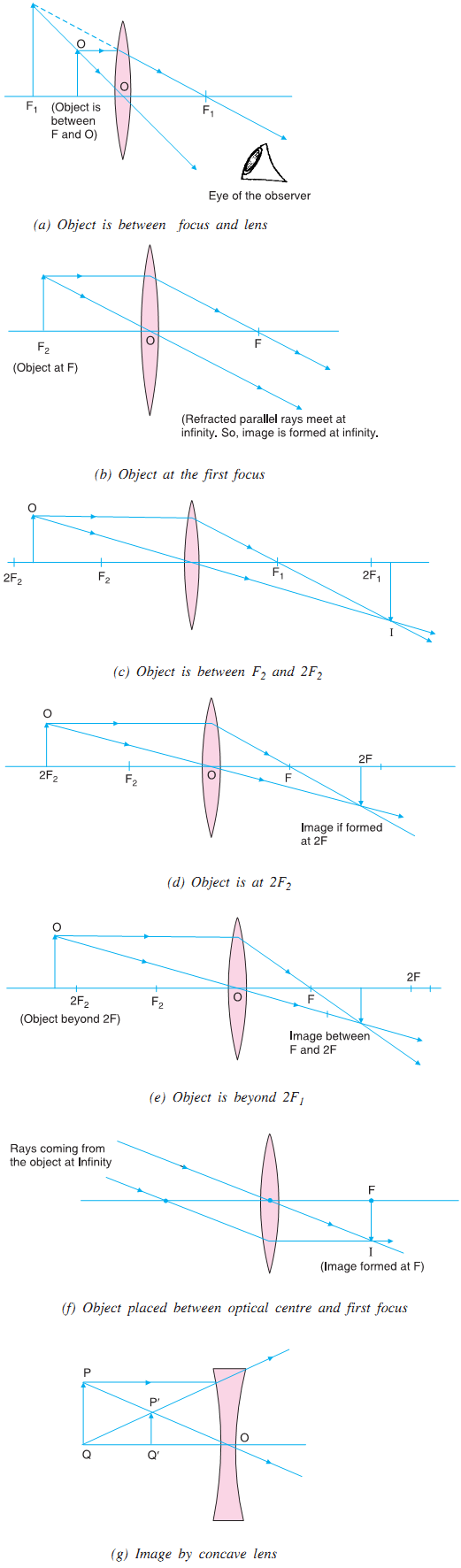All these images formed for different positions of object and nature of the image can be summarized as:

 S.No. Object Position Image Position Nature Size Convex Lens 1 Betwwen P & F Infront of lens Virtual & Erect Larger 2 At F At infinity Real & Inverted Enlarged 3 Between F & 2F Beyond 2F Real & Inverted Larger 4 At 2F At 2F Real & Inverted Same size 5 Beyond 2F Between F & 2F Real & Inverted Smaller 6 At infinity At F Real & Inverted Diminished Concave Lens 7 Anywhere Between P & F Virtual & Erect Smaller

### Sign Convention

In case of spherical lenses:

1. all distances in a lens are to be measured from optical centre of the lens
2. distances measured in the direction of incident ray are taken to be positive
3. distance opposite to the direction of incident ray are taken to be negative
4. the height of the object or image measured above the principal are taken positive whereas below it, are taken negative.

### Lens Formula

If the distance of object from the optical centre of the lens is u, distance of image from the optical centre is v and focal length of the lens is f, then the relationship between u, v and f for lens is:

1/f = 1/v - 1/u

This is called lens formula. Focal length for convex lens is positive, for concave lens it is taken negative.

### Magnification

In case of some lenses, the size of the image of an object is enlarged where as in some other cases it is diminished. The ratio of the size of the image to the size of the object for a particular lens remains constant for that lens. The ratio of the size of the image to that of the object is called as the magnification of the lens.

Magnification = size of image (I) / size of object (O)

m = I/O = v/u

### Dispersion of Light through Prism

A prism is a transparent medium bounded by any number of surfaces in such a way that the surface on which light is incident and the surface from which light emerges are plane and non-parallel

When white light or sun light passes through a prism it splits up into constituent colours. This phenomenon is called dispersion and arises due to the fact that refractive index of prism is different for different colours of light. So, different colours in passing through a prism are deviated through different angles. Rainbow, the most colourful phenomenon in nature, is primarily due to the dispersion of sunlight by rain drops suspended in air.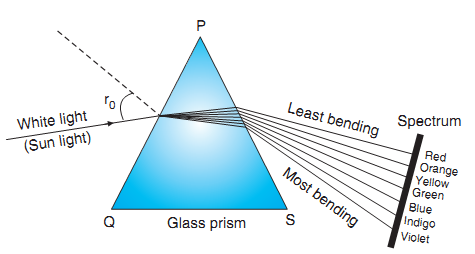### Eye and its Defects

In eye a convex lens forms real, inverted and diminished image at the retina. The lens can changes its convexity to form a suitable image as the distance between eye lens and retina fixed. The human eye is most sensitive to yellow-green light having wavelength 5550 Å, the least to violet 4000 Å and red 7000 Å.

The far and the near points for normal eye are usually taken to be at infinite and 25 cm respectively. It means a normal eye can see very distant objects clearly but near objects only if they are at a distance greater than 25 cm from the eye. The ability of eye to see objects from infinite distance to 25 cm is called power of accommodation.

If an object is at infinity, i.e., parallel beam of light enters the eye, the eye is least strained and said to relaxed or unstrained. However, if the object is at the least distance of distinct vision (= 25 cm), eye is under the maximum strain and visual angle is maximum. (The angle made by object at eye is called visual angle).

If image of the object does not form at retina the eye has some defects of vision.

Myopia

In this defect the distant objects are not clearly visible i.e., far point is at a distance lesser than infinity and hence image of distant object is formed before the retina. This defect is removed by using diverging (concave) lens. Myopia is also called short sightedness or near sightedness.Hyper metropia

It is also called long sightedness or far sightedness. In it the near objects are not clearly visible i.e. near point is at a distance greater than 25 cm. So the image of near object is formed behind the retina. This defect is removed by using converging lens.Presbypia

In this defect both near and far object are not clearly visible i.e., far point is lesser than infinity and near point greater than 25 cm. This can be removed either by using two separate spectacles one for myopia and other for hypermetropia or by using bifocal lens. It is an old age disease.

At old age ciliary muscles lose their elasticity so they can not change the focal length of eye lens effectively and eye losses its power of accommodation.

Astigmatism

It is due to imperfect spherical nature of eye lens. The focal length of eye lens is in two orthogonal directions become different so they can not see objects in two orthogonal directions simultaneously. This defect in direction can be removed by using cylindrical lens in a particular direction.